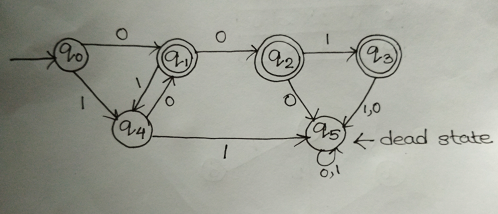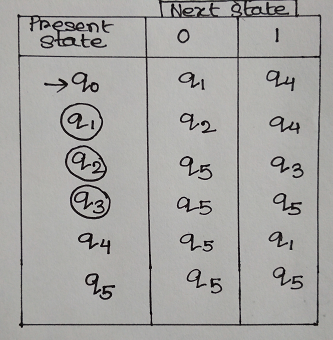# Question & Answer: Define a finite state machine (FSM) that recognizes words in the language (input alp…..

(1)

Write a regular expression for the language.

Don't use plagiarized sources. Get Your Custom Essay on
Question & Answer: Define a finite state machine (FSM) that recognizes words in the language (input alp…..
GET AN ESSAY WRITTEN FOR YOU FROM AS LOW AS \$13/PAGE

(2)

Define a finite state machine (FSM) that recognizes words in the language (input alphabet, states, start state, state transition table, and accept states). Include a state digraph for the FSM.

A: For alphabet {0,1}, all strings of length 3 or less that have more 0’s than 1’s

Solution:

Regular Expression:

A regular expression defines pattern of text or set of characters which is an alphabet set ( for example {0,1} for this question) to define particular language or regular language.

Finite State Machine:

A finite state machine is defined by 5 tuples: ( Q, , q0, F) where Q is the set of states, is the set of input alphabet,  is the transition function which maps Q X  -> Q, q0 is the start symbol, and F is the set of final states.

Regular Expression for the language will be:

001 + 100 + 010 + 00 + 0

As mentioned in the question, in the above solution the string length is 3 for the first 3 types ( 001, 100, 010) where number of 0’s are greater than number of 1’s. The string size is less than 3 for 00 and 0 where no 1 appears to fulfill the condition.

FSM for the above language is:

Here Q = { q0, q1, q2, q3, q4, q5}

= {0,1}

q0 is the initial state.

F = {q1, q2, q3}In the above diagram, the strings 010,100, 001, 00 and 0 is accepted by the FSM( keeping the string length <= 3). A dead state q5  is initialized so that if the FSM is given more input which are not accepted, then the FSM goes into dead state.

State Diagram: